## RD Sharma Class 8 Solutions Chapter 6 Algebraic Expressions and Identities Ex 6.3

These Solutions are part of RD Sharma Class 8 Solutions. Here we have given RD Sharma Class 8 Solutions Chapter 6 Algebraic Expressions and Identities Ex 6.3

Other Exercises

Find each of the following products (1-8)
Question 1.
5x2 x 4x3
Solution:
5x2 x 4x3 = 5 x 4 x x2 x x3
= 20x2 + 3 = 20xs

Question 2.
3a2 x 4b4
Solution:
-3a2 x 4b4 = -3 x 4 x a2b4
= -12a2b4

Question 3.
(-5xy) x (-3x2yz)
Solution:
(-5xy) x (-3x2yz)
= (-5) x (-3)xy x x2yz
= 15x1 + 2xy1+ 1z= 15x3y2z

Question 4.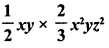Solution: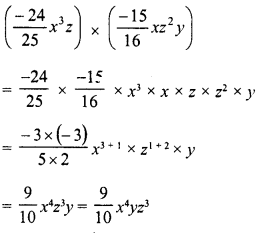Question 5.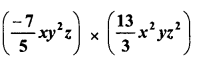Solution: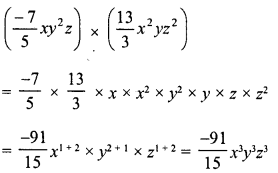Question 6.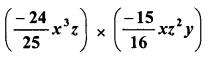Solution:

Question 7.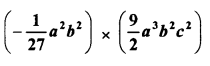Solution: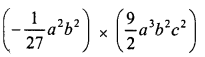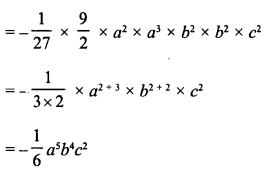Question 8.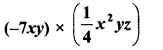Solution: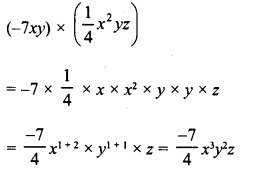Find each of the following products : (9-17)

Question 9.
(7ab) x (-5ab2c) x (6abc2)
Solution:
(7ab) x (-5ab2c) x (6abc2)
= 7 x (-5) x 6 x a x a x a x b x b2 x b x c x c2
=-210 x a1+1+1 x b1+2+1x c1+2
=-210 x a3b4c3

Question 10.
(-5a) x (-10a2) x (-2a3)
Solution:
(-5a) x (-10a2) x (-2a3)
= (-5) (-10) (-2) x a x a2 x a3
= -100a1 + 2 + 3 = -100a6

Question 11.
(-4x2) x (-6xy2) x (-3yz2)
Solution:
(-4x2) x (-6xy2) x (-3yz2)
= (-4) x (-6) x (-3) x2 x x x y2 x y xz2
= -72x2+1 x y2+1 x z2
= 72x3y3z3

Question 12.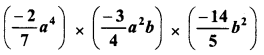Solution: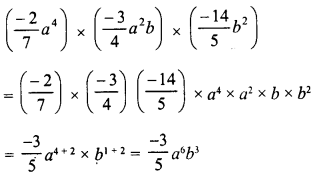Question 13.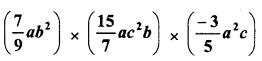Solution: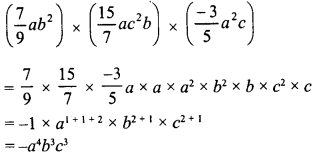Question 14.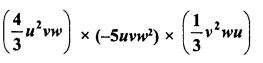Solution: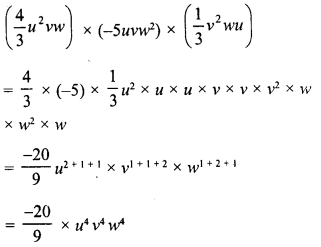Question 15.Solution: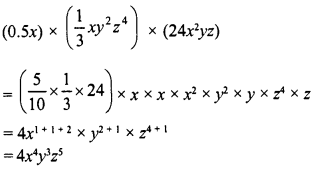Question 16.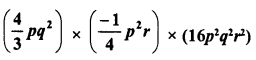Solution: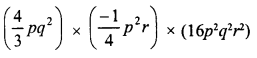Question 17.
(2.3xy) x (0.1x) x (0.16)
Solution:
(2.3xy) x (0.1x) x (0.16)
= 2.3 x 0.1 x 0.16 x x x x x y
= 0.0368x1 +1 x y = 0.0368x2y

Express each of the following products as a monomials and verify the result in each case for x = 1 : (18 -26)

Question 18.
(3x) x (4x) x (-5x)
Solution: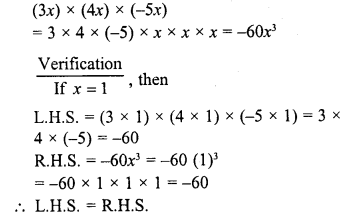Question 19.Solution:Question 20.
(5x4) x (x2)3 x (2x)2
Solution:
(5x4) x (x2)3 x (2x)2
= 5x4 x x2 x 3 x 2x x 2x
= 5x4 * x6 x 4x2 = 5 x 4 x x4 + 6 + 2
= 20x12
Verification:
L.H.S. = (5x4) x (x2)3 x (2x)2
= 5 x (1)4 x [(1)2]3 x (2 x 1)2
= 5 x 1 x (1)2 x 3x (2)2
= 5 x 16 x 22 = 5 x 1 x 4 = 20
R.H.S. = 20x12 = 20 (1)12 = 20 x 1 = 20
∴ L.H.S. = R.H.S.

Question 21.
(x2)3 x (2x) x (-4x) x 5
Solution:
(x2)3 x (2x) x (-4x) x (5)
= x2 x 3 X 2x X (-4x) X 5
= x6 x 2x x (-4x) x 5 = 2 x (-4) x 5x6+1 +1
= -40x8
Verification
L.H.S. = (x2)3 x (2x) x (-4x) x (5)
= (12)3 x (2 x 1) x (-4 x 1) x 5
= 1x 2 x (- 4) x 5 = 16 x 2 x (-4) x 5
= 1 x 2 x (-4) x 5 = -40
R.H.S. = -40x8 = -40 x (1)8
= -40 x 1 = -40
∴ L.H.S. = R.H.S.

Question 22.
Write down the product of -8x2y6 and – 20xy Verify the product for x = 2.5, y = 1.
Solution:
Product of -8x2y6 and -20xy
= (-8x2y6) x (-20xy)
= -8 x (-20) x2 x x x y6 x y = 160x2 + 1 x y6 + 1
= 160x3y3
Verification.
L.H.S. = (-8x2y6) x (-20xy)
= -8 x (2.5)2 x (1) x (-20 x 2.5 x 1)
= -8 x 6.25 x 1 x -20 x 2.5
= (-50) x (-50) = 2500
R.H.S. = 160 x = 160 (2.5)3 x (1)7
= 160 x 15.625 x 1 =2500
∴ L.H.S. = R.H.S.

Question 23.
Evaluate : (3.2x6y3) x (2.1x2y2) when x = 1 and y = 0.5.
Solution:
3.2x6y3 x 2.1x2y2
= 3.2 x 2.1 x x6+2 x y3+2
= 6.72x8y5 = 6.72 x (1)8 x (0.5)5
= 6.72 x 1 x 0.03125
= 0.21

Question 24.
Find the value of (5x6) x (-1.5x2y3) x (-12xy2) when x = 1, y = 0.5.
Solution:Question 25.
Evaluate : (2.3a5b2) x (1.2a2b2) when a = 1, b = 0.5.
Solution:Question 26.
Evaluate : (-8x2y6) x (-20xy) for x = 2.5 and y = 1.
Solution:Express each of the following products as a monomials and verify the result for x = 1,y = 2: (27-31)

Question 27.
(-xy3) x (yx3) x (xy)
Solution:
(-xy3) x (yx3) x (xy)
= -x x xx x x yx y x y = -x1 + 3 + 1 x y3 + 1 + = -x5y5
Verification:
L.H.S. = (-xy3) x (yx3) x (xy)
= (-1 x 23) x [2 x (1)3] x (1 X 2)
= (-1 x 8) x (2 x 1) x (1 x 2)
= -8 x 2 x 2 = -32
R.H.S. =-x5y5  = -(1)5 (2)5
= -1 x 32 =-32
∴ L.H.S. = R.H.S.

Question 28.Solution: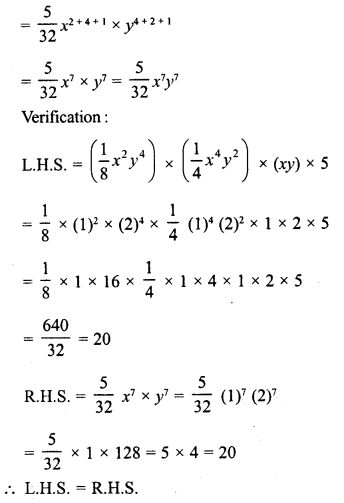Question 29.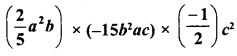Solution:Question 30.Solution: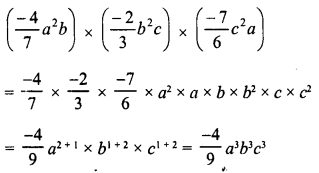Question 31.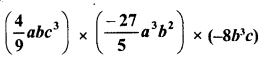Solution: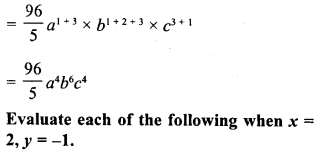Question 32.Solution: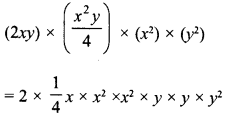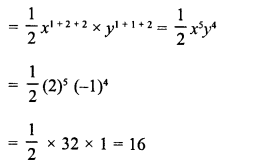Question 33.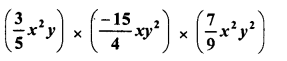Solution: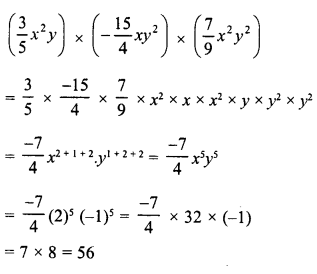Hope given RD Sharma Class 8 Solutions Chapter 6 Algebraic Expressions and Identities Ex 6.3 are helpful to complete your math homework.

If you have any doubts, please comment below. Learn Insta try to provide online math tutoring for you.